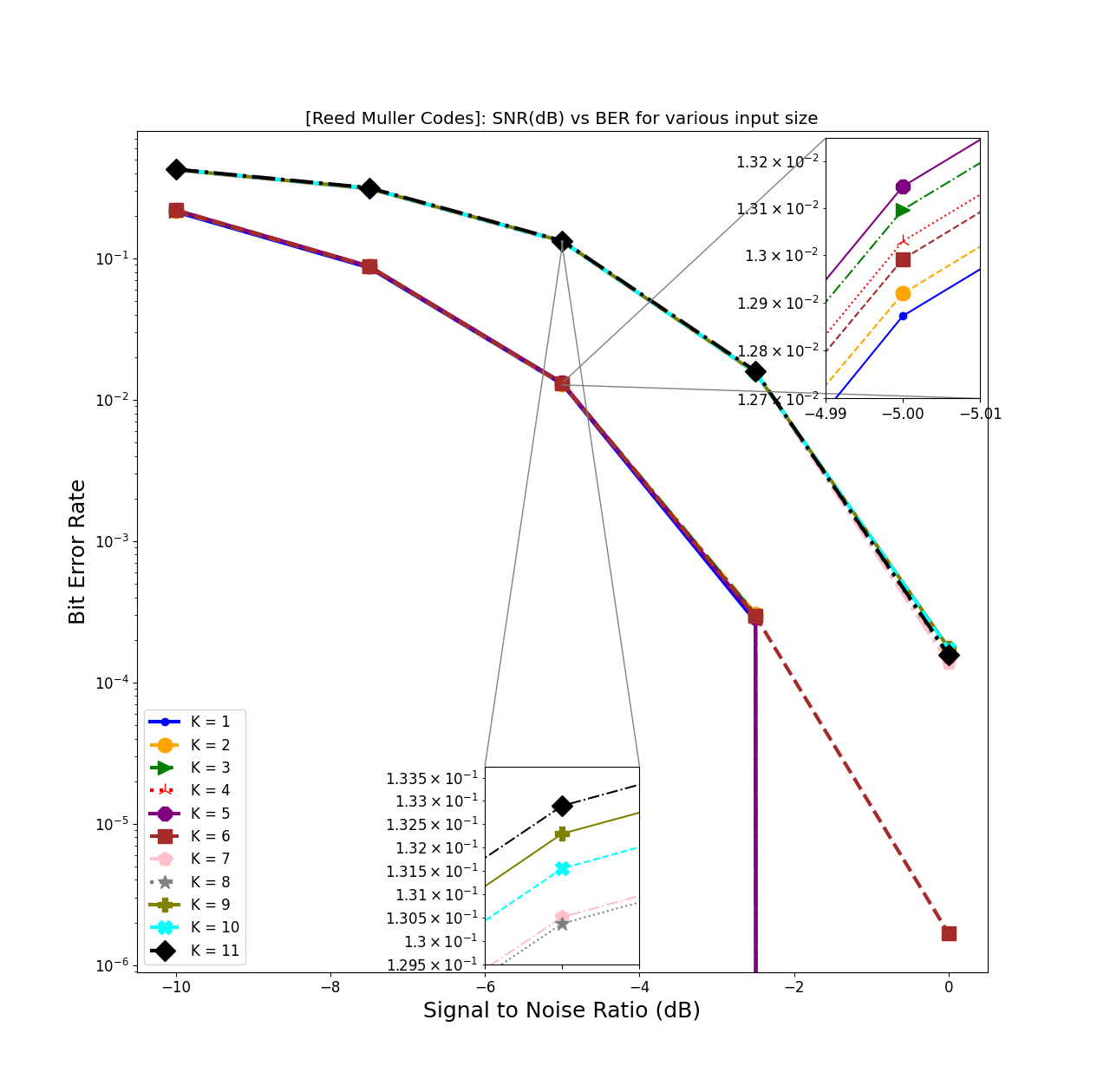# Reed Muller Codes

5G is required to transport payload of various sizes. The very small payload sizes are forward error corrected using Reed Muller codes which are designed to provide protection to control information bit with length between 3 to 11. The figure-1 demonstrates the bit error rate (BER) vs signal to noise ratio (SNR) performance of Reed Muller codes for AWGN channels. The details of this are provided in tutorial-x.Important

• The Reed Muller Codes are used in 5G to encode downlink control information bits of length 3 to 11. The length of the codeword is fixed to 32.

## Reed Muller Encoder 5G

This coder can process multiple batches and codewords simultaneous shown in following three examples:

When input is a vector:

K            = 5  # Number of Information bits
# Generate information bits
bits         = np.random.randint(2, size = K)
codeword     = ReedMullerEncoder5G()(bits) # Encode the information bits


When input is a 2D array:

K            = 5  # Number of Information bits
numBatches   = 20 # Number of Batches
# Generate information bits
bits         = np.random.randint(2, size = (numBatches, K))
codeword     = ReedMullerEncoder5G()(bits) # Encode the information bits


When input is a tensor:

K            = 5  # Number of Information bits
numBatches   = 20 # Number of Batches
numCodewords = 2  # umber of codewords
# Generate information bits
bits         = np.random.randint(2, size = (numBatches, numCodewords, K))
codeword     = ReedMullerEncoder5G()(bits) # Encode the information bits


The details about the input-output interface of the Reed Muller modules is provided below.

class toolkit5G.ChannelCoder.ReedMullerEncoder5G(dtype=None)[source]

This module implements a 5G standards complaint Encoder for Reed Muller Codes. The module encodes the input bits to a codeword of length 32. The details of Encoding procedure are provided in section 5.3.3 of 38.212 specifications [3GPPTS38211_RM].

Parameters:

dtype (np.dtype) – Defines the datatype of the decoded bits. Default value is np.int8.

Input:

inBits ([…,k], np.int32) – Defines the information bits to be encoded.

Warning

The number of input bits, k $$\in \{3,4,...,11\}$$

Output:

[…,32], dtype – 2+D tensor containing encoded bits.

Raises:
• ValueError – [Error-ReedMullerEncoder5G]: ‘input Bits’ must be an numpy array of integers/floats!

• ValueError – [Error-ReedMullerEncoder5G]: number of ‘input Bits’ for encoding shall be positive non zero number less than 12!

• ValueError – [Error-ReedMullerEncoder5G]: ‘input Bits’ for encoding is a zero dimensions array!

• ValueError – [Error-ReedMullerDecoder]: ‘dtype’ must be integer type!

• ValueError – [Error-ReedMullerDecoder]: ‘k’ must be integer!

property dtype

Defines the datatype of the decoded bits. Default value is np.int8.

property k

Number of information bits after decoding.

## Reed Muller Decoder 5G

The following example demonstrate the way to use the Reed Muller for decoding the codeword. Note that, the hardIn must be set to False if the input codeword contains the log likelihood ratio (LLR) values of each bit and True otherwise.

hard_in = False
decoder = ReedMullerDecoder5G(K, hard_in)
rxBits  = decoder(llrEst)


The details about the input-output interface of the Reed Muller modules is provided below.

class toolkit5G.ChannelCoder.ReedMullerDecoder5G(k, hardIn=False, dtype=<class 'numpy.int8'>)[source]

This module implements the 5G standards complaint Decoder for the Reed Muller Codec specified in section 5.3.3 of [3GPPTS38211_RM]. It accepts both hard and soft inputs which are to be configured via hardIn.

Parameters:
• k (int) – Number of information bits after decoding $$\in \{3,4,\dots,11\}$$

• hardIn (bool) – Set to true if the codeword (input) is binary. Default value is False.

• dtype (np.dtype) – Defines the datatype of the decoded bits. Default value is np.int8.

Input:

codeword ([…,32], np.float32) – Defines the codeword to be decoded.

Output:

[…,k], dtype – 2+D tensor containing hard-decided estimates of all k information bits.

Raises:
• TypeError – [Error-ReedMullerEncoder5G]: ‘codeword’ must be an numpy array of integers/floats!

• ValueError – [Error-ReedMullerEncoder5G]: The hard ‘input Bits’ can be either 0 or 1!

• Exception – [Error-ReedMullerEncoder5G]: number of ‘codeword Bits’ for decoding should be 32!

• Exception – [Error-ReedMullerEncoder5G]: ‘codeword’ for decoding is a zero dimensions array!

• ValueError – [Error-ReedMullerDecoder]: ‘dtype’ must be integer type!

• ValueError – [Error-ReedMullerDecoder]: ‘k’ must be integer!

• ValueError – [Error-ReedMullerDecoder]: ‘hardin’ must be bool!

property dtype

Defines the datatype of the decoded bits. Default value is np.int8.

property hardIn

Set to true if the codeword (input) is binary. Default value is False.

property k

Number of information bits after decoding.

References:
[3GPPTS38211_RM] (1,2)

3GPP TS 38.211 “Physical channels and modulation (Release 17)”, V17.1.0 (2022-03).